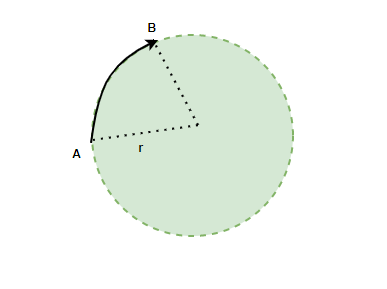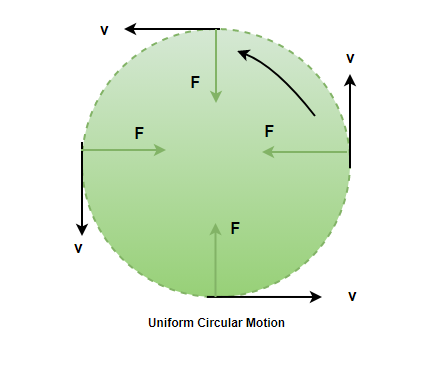Open in App
Not now

# Dynamics of Circular Motion

• Last Updated : 14 Jul, 2021

There are lots of examples around us in daily lives where bodies perform circular motions every day. From the hands of the clock to a car turning on a banked road. All these are examples of circular motion. This motion can be classified into two categories – uniform circular motion and non-uniform circular motion. It is essential to know the dynamics and the equation of the body performing the circular motion. These dynamics allow us to analyze these motions and calculate the statistics related to the behavior of the body in such motion.

### Circular Motion

An object moving in a circular trajectory around a point is said to be performing a circular motion. For example — a car turning on the road is performing a circular motion around some center. The circular motion of continued is periodic in nature. Periodic motions are the motions that repeat themselves after a certain period of time. Based on the velocity and the acceleration, this motion can be classified into two categories –

1. Uniform Circular Motion- When a body moves at constant speed in a circular motion along the circumference of the circular trajectory, it is called Uniform Circular Motion.
2. Non-Uniform circular motion- When a body moves in a circular motion along the circumference of a circular trajectory in such a way that its speed keeps changing. It is called a non-uniform circular motion.

### Dynamics of Circular Motion

In this motion, the body is moving at a constant speed. Let’s say the radius of the circular trajectory on which the body is moving is “r”, and the speed of the body is v m/s. The figure shows the body going from point A to point B in time “t”. The length of the arc from point A to point B is denoted by “s”. In this, the angle covered by the object is given by,Angular velocity of the body is defined as the rate of change of angle. It’s similar to velocity in the case of straight-line motion.  It is denoted by the Greek symbol.Using the relation given above for the angle covered.S is the length of arc which is the distance covered by the body,

V =, where v is the speed of the body.

Substituting the value in the equation,### Uniform Circular motion

Bodies have a tendency to move in a straight line. For the bodies making a circular motion at a constant speed, there must be some force that keeps them on a circular path. Such a force is called the centripetal force. The reaction of this force is called centrifugal force. This means that both these forces are equal and opposite in direction.Centrifugal force is given by,It is known thatSubstituting this relation into the equation,### Sample Problems

Question 1: Find the angular velocity of a body that is moving at a speed of 20m/s in a circle of radius 5 m.

The formula for angular velocity is given by,Given: v = 20m/s and r = 5 m.

Plugging the values into the equation,Question 2: Find the angular velocity of a body that is moving at a speed of 10m/s in a circle of radius 2 m.

The formula for angular velocity is given by,Given: v = 10m/s and r = 2 m.

Plugging the values into the equation,Question 3: Find the force acting on a particle of mass 2Kg moving in a circle of radius 2 m at a speed of 5 m/s.

The formula for centripetal force is given by,Given: v = 5 m/s, r = 2 m and m = 2Kg

Plugging the values into the equation,⇒F = 25N

Question 4: Find the force acting on a particle of mass 5Kg moving in a circle of radius 4 m at an angular speed of 20 rad/s.

The formula for centripetal force is given by,Given:, r = 2 m and m = 2Kg

Plugging the values into the equation,⇒F = 1600 N

Question 5: An insect moves in a circle of 2 m radius and completes 10 revolutions per second. Find the angular velocity, linear velocity, and acceleration.

The body moves at 10 revolutions per second.The angular velocity isThe linear velocity is given by,Given: r = 2 m⇒ v = (2)(20)

⇒ v=Acceleration will be given by,

a =⇒ a =⇒a =Question 6: An insect moves in a circle of 4 m radius and completes 20 revolutions per second. Find the angular velocity, linear velocity, and acceleration.

The body moves at 20 revolutions per second.The angular velocity isThe linear velocity is given by,Given: r = 4 m⇒ v = (4)(40)

⇒ v=Acceleration will be given by,

a =⇒ a =⇒a =My Personal Notes arrow_drop_up